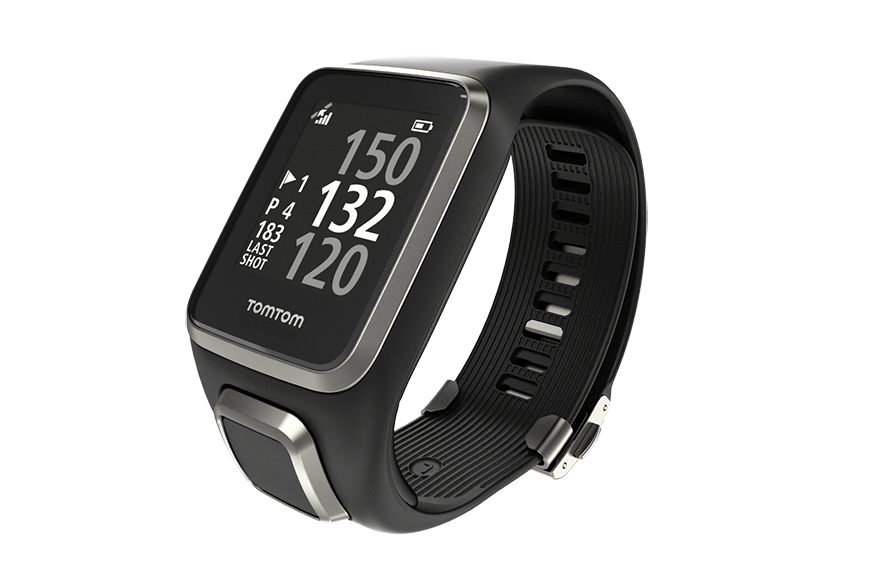iSoul In the beginning is reality.

# Measurement of space and time

The various ways of measuring space and time are parallel.

Measuring space:

1. A ruler measures length, that is, the distance between two points in space (A to B).
2. An ruler turned upside-down measures length backwards (B to A).
3. A tripmeter measures the travel distance of a vehicle trip.
4. An odometer measures the cumulative travel distance.5. A measuring wheel measures the travel distance of a wheel being pushed.
6. A road map measures travel distance of a standard vehicle. See Geodistance.

Measuring time:

1. A stopwatch measures time, that is, the duration between two points in time (A to B).
2. A timer measures the time counting down from a set time, i.e., backwards (B to A).
3. A GPS watch or time clock measures the duration of an activity, such as running or working.4. A GPS watch (or smartphone app) measures cumulative travel time (or flight time).
5. A measuring wheel with a stopwatch measures the travel time of a wheel being pushed.
6. A clock measures travel time synchronized with a standard motion.

Note that #2 shows time can be measured backwards. Space and time can both be counted up or counted down. There’s nothing magical about it.